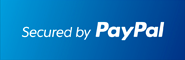** New: ECONOMICS , DIFFERENTIAL EQUATIONS **         ** SPECIALS for bulk apps **         ** Bestsellers: CALCULUS , SAT , PHYSICS , CHEM , STOCKS & PORTFOLIOS , ALGEBRA **# #1 Numerical Analysis Solver for the TI-89 Calculator

## Solve Numerical Analysis problems Step by Step.

### Works With TI92 Plus TI89 TI89 Titanium Voyage 200At the end of the PayPal checkout, you will be sent an email containing your key and software.Description

• The most comprehensive Numerical Analysis APP for calculators.
• Step by Step Numerical Integration using Simplex 1/3 and 3/8,
Midpoint, Rectangle or Trapezoid Methods.
• Step by Step Interpolation using Conventional way or Lagrange Polynomials
• Step by Step Solving Linear Systems: Gauss Elimination
• Step by Step Simplex Algorithm
• Step by Step Square Root Matrix
• Step by Step Markov Chains
• Step by Step Regression Analysis and Data Analysis
• Step by Step Bisection, Newton and Fixpoint Methods
• Step by Step Quadratic Formula and Completing the Square
• Step by Step Fourier and Taylor Series Analysis
• Step by Step Partial Fractions
• Step by Step RREF : Reduced Row Echelon Method
• Step by Step Eigenvector and Eigenvalue
• Step by Step Fixpoint Method
• Step by Step Newton, Bisection Methods
• Step by Step Gauss Quadrature
• Any type of Statistical Regression
• Numerical Differentiation using the
direct/symmetric/forward/backward
differential quotient, also applied to partial derivatives.
• Solve Differential Equations numerically using Euler, Improved Euler with predictor step, Ralston, Heun, Runge Kutta or Midpoint Method
• Regula Falsi, False Position
• Gauss Seidel Method
• Pi Approximation
• Orthogonal Polynomials
• Simpson Method
• Tangent and Secant Lines
• Cholesky, LU and QR Factorization